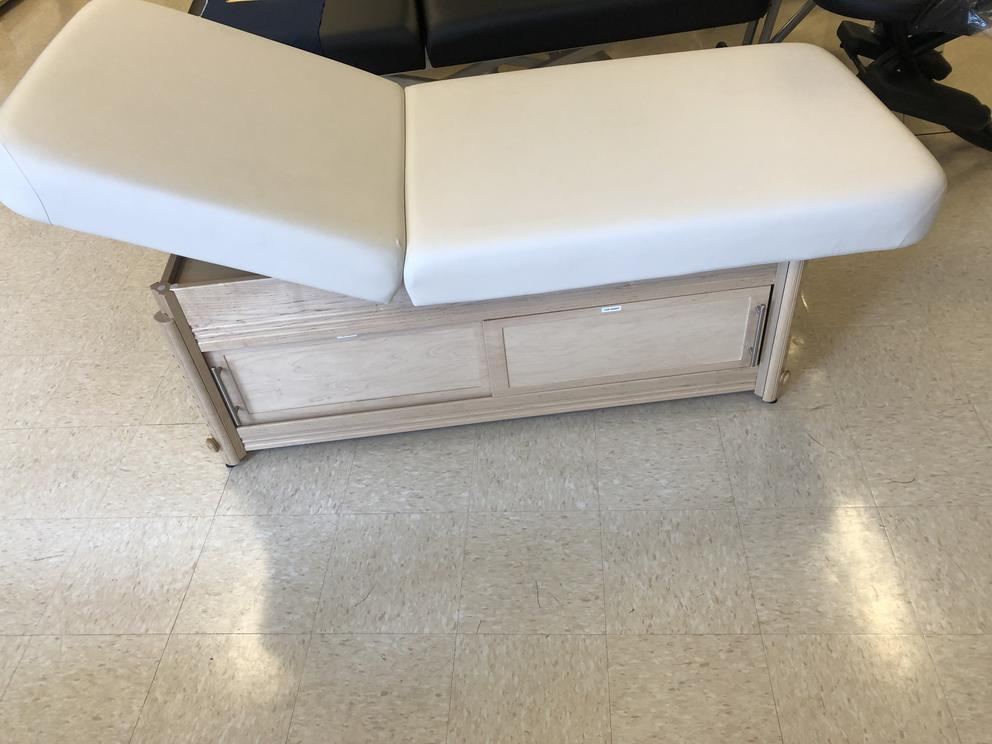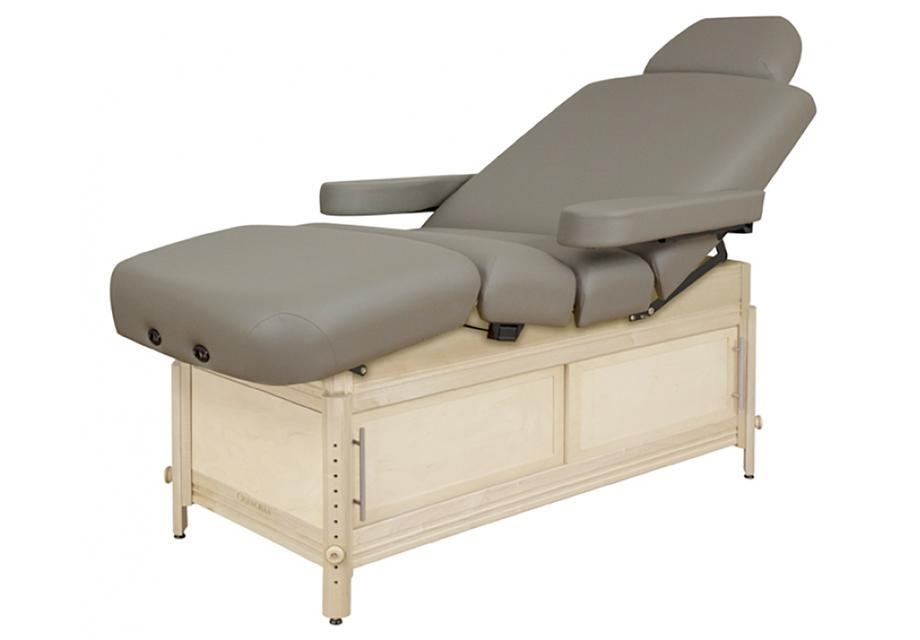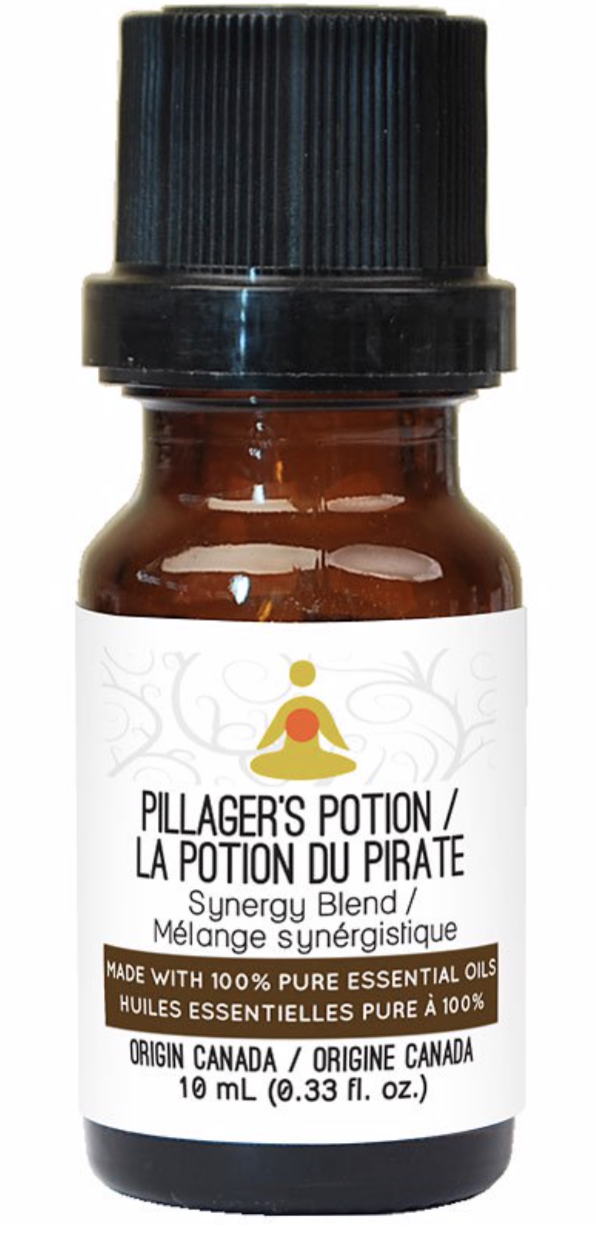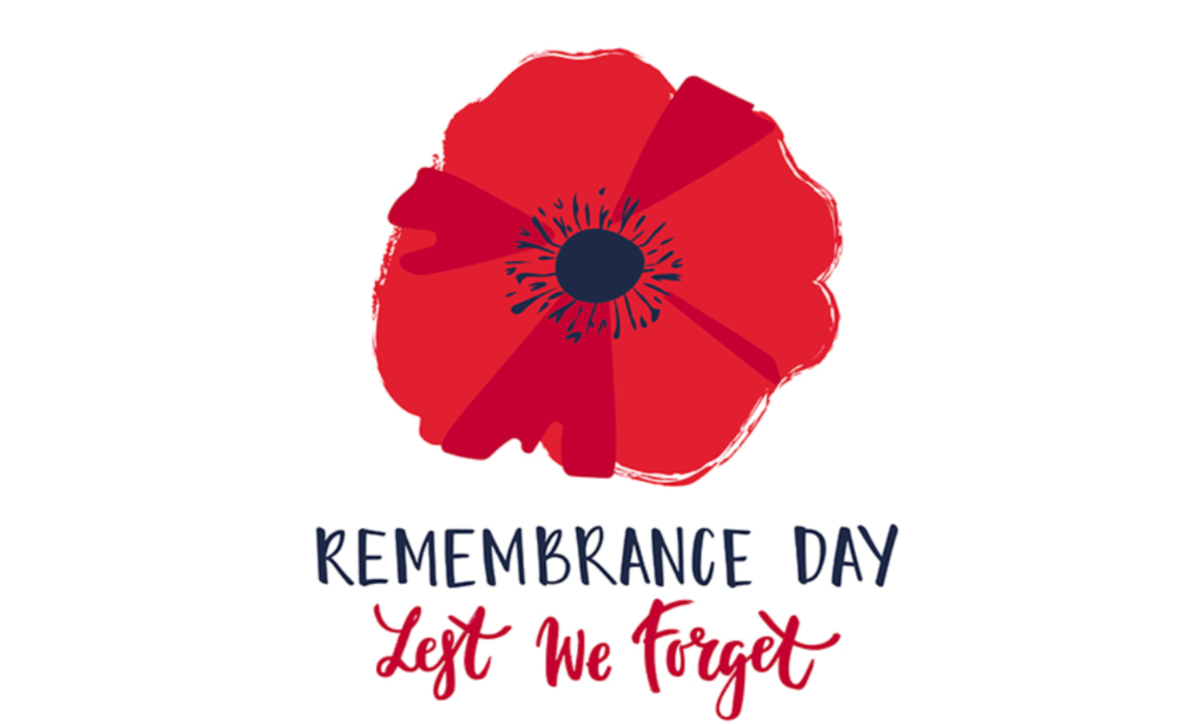## MTSO November News - time to keep warm!It's winter, and time to help your clients stay healthy, warm and cozy. And we have just the products you need! We're featuring Hydrocollators, Towel Warmers (with UV) and of course, Cleaners. Plus: Pillager's Potion, based on the famous 4 thieves standard. Also, we're featuring special pricing on Spa Tables with back UpLift. Scroll down for more....

 table div table+table+table+table div table{width:100%;padding:0}table div table+table+table+table div table img{width:96.23%;padding:0;float:none}table div table+table+table+table div table td{width:100%;padding:0 1.88% 18px}/* styles */# Hydrocollators with heating packs

The benefits of moist heat therapy over dry heat are well-established for pain relief. Immerse heat pack in Hydrocollator Heating unit to heat, then remove, wrap in its terry cover and apply to treatment area. It provides up to 30 minutes of deep-felt, soothing, moist heat. Cost effective, each heat pack unit provides hundreds of treatments. Your client's treatment will progress much faster by stimulating circulation to help remove toxins and increase the supply of rich, oxygenated nutrients that are available to damaged tissues.
Choose standard or large - both come with heat packs.

 table div table+table+table+table+table+table+table+table div table{width:100%;padding:0}table div table+table+table+table+table+table+table+table div table img{width:96.23%;padding:0;float:none}table div table+table+table+table+table+table+table+table div table td{width:100%;padding:0 1.88% 18px}/* styles */## STATIONARY SPA TABLES with back UPLIFTStationary Spa UpLift was \$1200 now \$1000 (cream only)Adjustable Spa UpLift was \$1350, now \$1200 (black only)
 /* styles */ Click on the image for more info on each of these amazing high quality stationary spa tables with uplift back. The Lift Assist Backrest Top allows you to easily and quietly adjust the incline of your table so that you can perform a wider range of procedures. Since the client doesn't even have to move during the adjustment process, the flow of the treatment session is maintained, and the client can remain on the table. The secret is the hydraulic gas cylinders that you control with easy to reach finger controls, putting the power to make table top adjustments at your fingertips.
 table div table+table+table+table+table+table+table+table+table+table+table+table div table{width:100%;padding:0}table div table+table+table+table+table+table+table+table+table+table+table+table div table img{width:96.23%;padding:0;float:none}table div table+table+table+table+table+table+table+table+table+table+table+table div table td{width:100%;padding:0 1.88% 18px}/* styles */The Pillagers Potion is a universal blend from the standard based on the 4 Thieves blend.

# Support your Immune System with Pillager's Potion

We're featuring Pillager's Potion for immune system support during "cold & flu season". It's an easy & pleasant blend to diffuse, & also very supportive applied during massage (1-2 drops at a time in about 1tsp of oil or lotion) to lymphatic drainage areas, & aching muscles. It's warming
& decongestive to all body systems.

 table div table+table+table+table+table+table+table+table+table+table+table+table+table+table+table div table{width:100%;padding:0}table div table+table+table+table+table+table+table+table+table+table+table+table+table+table+table div table img{width:96.23%;padding:0;float:none}table div table+table+table+table+table+table+table+table+table+table+table+table+table+table+table div table td{width:100%;padding:0 1.88% 18px}/* styles */# NEW! Towel Warmers with UV included.

UV is particularly good for keeping towels sanitized during the warming process until they are applied to body.
Our warmer heats and sanitizes about 24 towels at a time, so you'll always be good to go.

 table div table+table+table+table+table+table+table+table+table+table+table+table+table+table+table+table+table+table div table{width:100%;padding:0}table div table+table+table+table+table+table+table+table+table+table+table+table+table+table+table+table+table+table div table img{width:96.23%;padding:0;float:none}table div table+table+table+table+table+table+table+table+table+table+table+table+table+table+table+table+table+table div table td{width:100%;padding:0 1.88% 18px}/* styles */table div table+table+table+table+table+table+table+table+table+table+table+table+table+table+table+table+table+table+table div table,table.module-18{width:37.74%;float:left;padding:0}table div table+table+table+table+table+table+table+table+table+table+table+table+table+table+table+table+table+table+table div table a{border:0 none;text-decoration:none}table div table+table+table+table+table+table+table+table+table+table+table+table+table+table+table+table+table+table+table div table img{width:100%!important;border:0 none;text-decoration:none}table div table+table+table+table+table+table+table+table+table+table+table+table+table+table+table+table+table+table+table div table td{width:100%;padding:0 20px 20px 0}/* styles */ At Massage Therapy Supply Outlet we promote cleaning supplies that are safe, non-toxic, environmental and won't harm vinyl. Click the image to view our recommended selection.
 table div table+table+table+table+table+table+table+table+table+table+table+table+table+table+table+table+table+table+table+table div table{width:100%;padding:0}table div table+table+table+table+table+table+table+table+table+table+table+table+table+table+table+table+table+table+table+table div table img{width:96.23%;padding:0;float:none}table div table+table+table+table+table+table+table+table+table+table+table+table+table+table+table+table+table+table+table+table div table td{width:100%;padding:0 1.88% 18px}/* styles */As always, we will pause our commerce on Remembrance Day, Monday the 11th.
 table div table+table+table+table+table+table+table+table+table+table+table+table+table+table+table+table+table+table+table+table+table+table div table{width:100%;padding:0}table div table+table+table+table+table+table+table+table+table+table+table+table+table+table+table+table+table+table+table+table+table+table div table img{width:96.23%;padding:0;float:none}table div table+table+table+table+table+table+table+table+table+table+table+table+table+table+table+table+table+table+table+table+table+table div table td{width:100%;padding:0 1.88% 18px}/* styles */## Order online for delivery or pickup

Browse and buy from an extensive collection at our online store, always open, 24/7/365. Edmonton store and showroom: 10:00 - 6:00 Mon-Fri, 10:00-5:00 Sat.

 table.module-23{width:51.32%;padding:0}table div table+table+table+table+table+table+table+table+table+table+table+table+table+table+table+table+table+table+table+table+table+table+table+table div table{width:51.32%;float:none;margin-left:auto;margin-right:auto;padding:0}table div table+table+table+table+table+table+table+table+table+table+table+table+table+table+table+table+table+table+table+table+table+table+table+table div table a{border:0 none;text-decoration:none}table div table+table+table+table+table+table+table+table+table+table+table+table+table+table+table+table+table+table+table+table+table+table+table+table div table img{width:100%!important;border:0 none;text-decoration:none}table div table+table+table+table+table+table+table+table+table+table+table+table+table+table+table+table+table+table+table+table+table+table+table+table div table td{width:100%;padding:0}/* styles */
 Like   Tweet   Pin   in